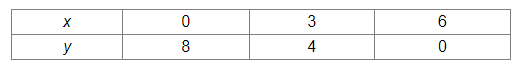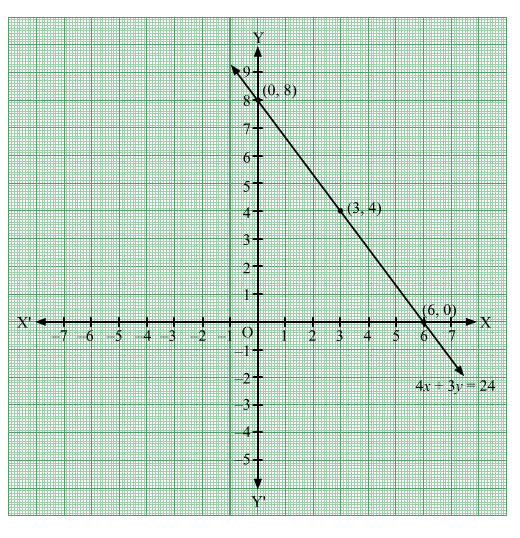# Draw the graph of the line 4x + 3y = 24.

Question:

Draw the graph of the line 4x + 3y = 24.
(i) Write the coordinates of the points where this line intersects the x-axis and the y-axis.
(ii) Use this graph to find the area of the triangle formed by the graph line and the coordinate axes.

Solution:

$4 x+3 y=24$

$\Rightarrow 3 y=-4 x+24$

$\Rightarrow y=\frac{-4 x+24}{3}$

When $x=0, y=\frac{-4 \times 0+24}{3}=\frac{0+24}{3}=\frac{24}{3}=8$

When $x=3, y=\frac{-4 \times 3+24}{3}=\frac{-12+24}{3}=\frac{12}{3}=4$

When $x=6, y=\frac{-4 \times 6+24}{3}=\frac{-24+24}{3}=\frac{0}{3}=0$

Thus, the points on the line 4x + 3y = 24 are as given in the following table:Plotting the points (0, 8), (3, 4) and (60) and drawing a line passing through these points, we obtain the graph of of the line 4x + 3y = 24.(i) It can be seen that the line 4x + 3y = 24 intersects the x-axis at (6, 0) and y-axis at (0, 8).

(ii) The triangle formed by the line and the coordinate axes is a right triangle right angled at the origin.

$\therefore$ Area of the triangle $=\frac{1}{2} \times 6 \times 8=24$ square units